Function Repository Resource:

# ConfusionMatrixToClassifierMeasurementsObject

Convert a confusion matrix into a ClassifierMeasurementsObject from which statistics can be derived

Contributed by: Seth J. Chandler
 ResourceFunction["ConfusionMatrixToClassifierMeasurementsObject"][m,classes] converts a numeric confusion matrix m into a ClassifierMeasurementsObject where classes are a list of the classes.

## Details

If the confusion matrix contains more columns than rows, it will be assumed that the final column is for indeterminate cases; these indeterminate cases will not be considered when generating the ClassifierMeasurementsObject.
The input confusion matrix should follow the convention that the actual class is on the rows and the machine's classification is on the columns.

## Examples

### Basic Examples (2)

Create a ClassifierMeasurementsObject out of a confusion matrix where the classes are "healthy" and "sick":

 In:=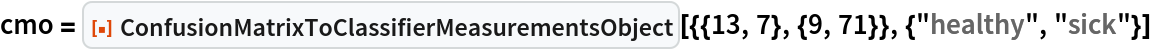Out=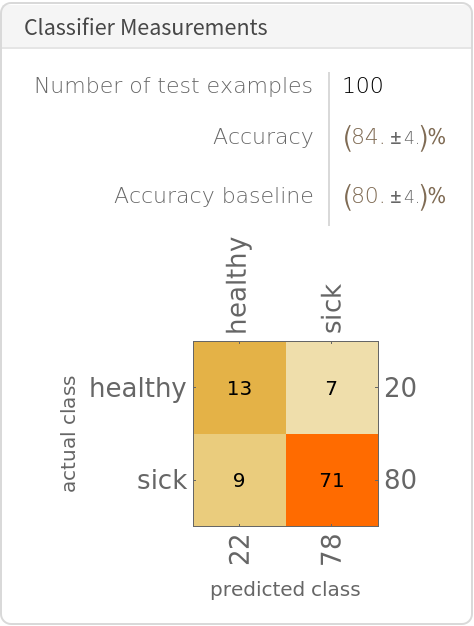Show the properties of the object generated by the confusion matrix:

 In:=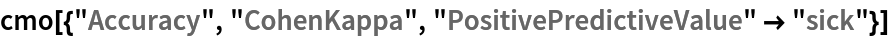Out=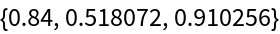### Scope (3)

Show a confusion matrix in which the last column represents indeterminate cases and thus is dropped:

 In:=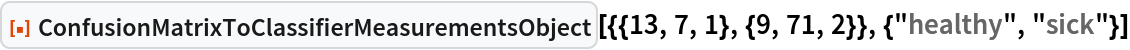Out=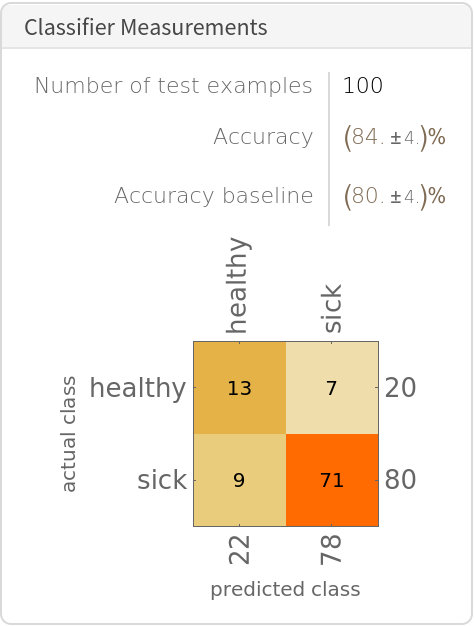A confusion matrix with five classes:

 In:=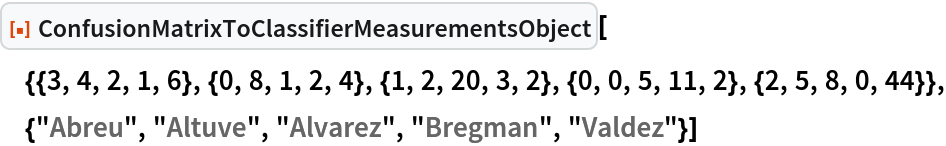Out=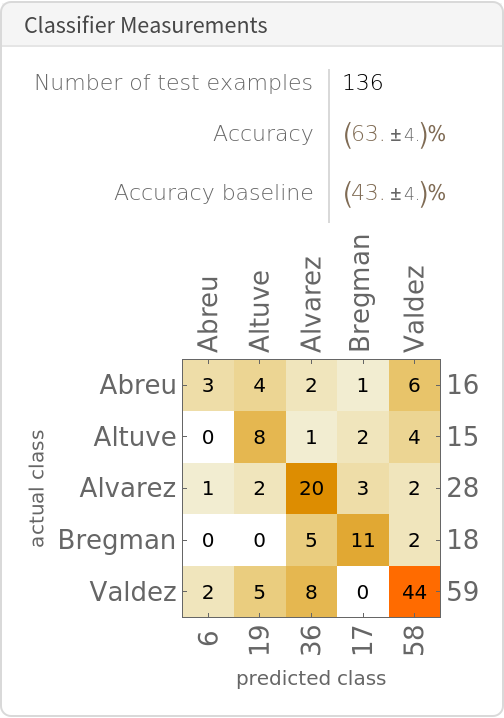Numeric properties of a ClassifierMeasurementsObject are available:

 In:=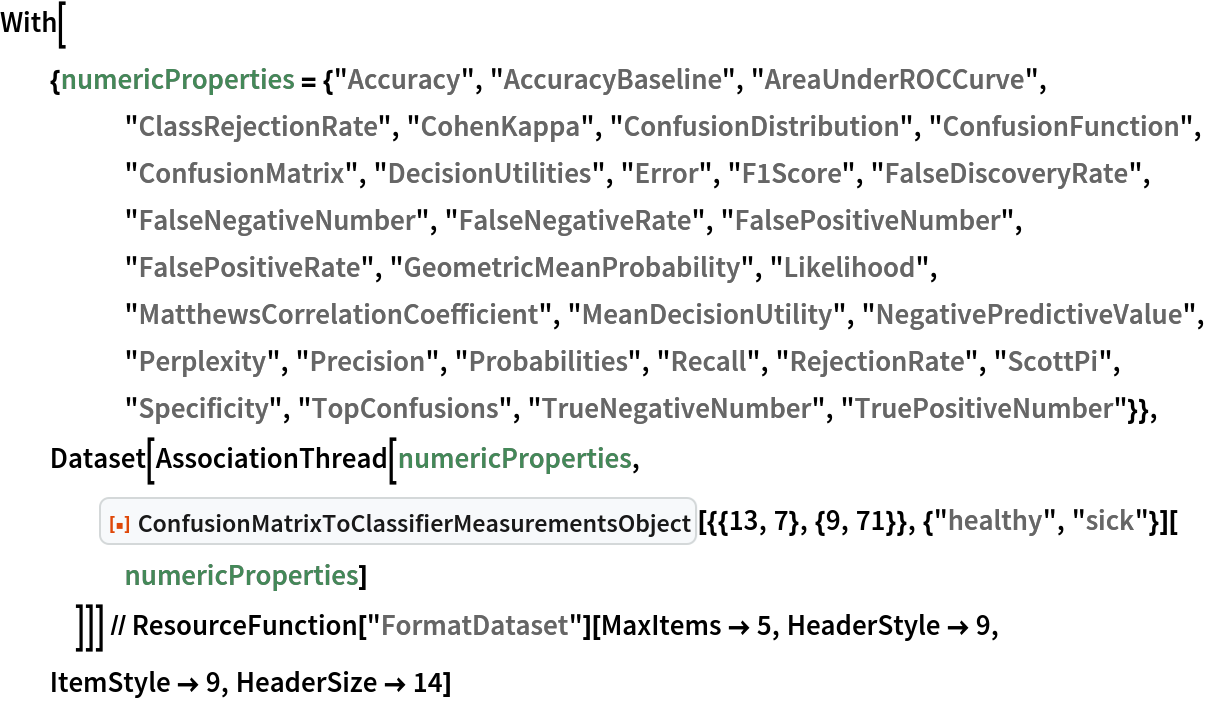Out=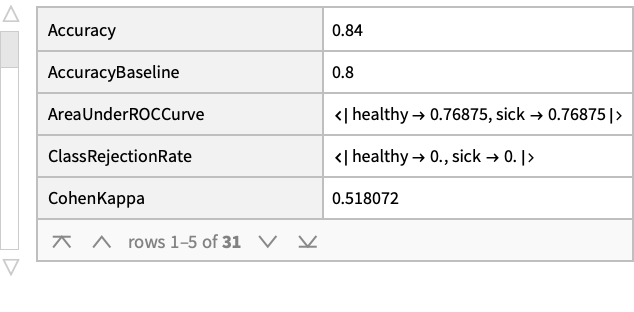Properties that produce plots are also available:

 In:=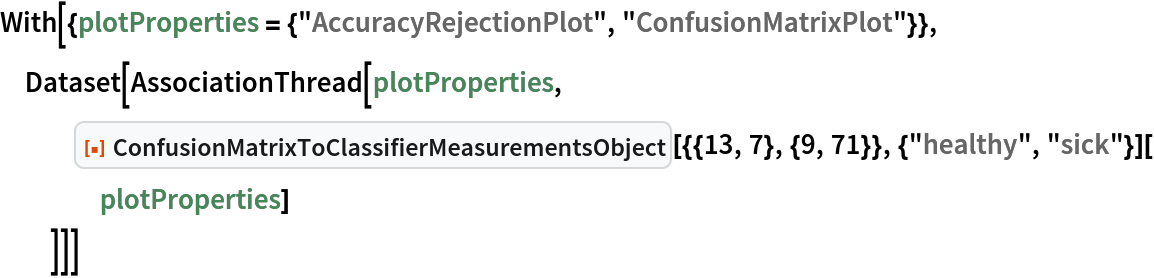Out=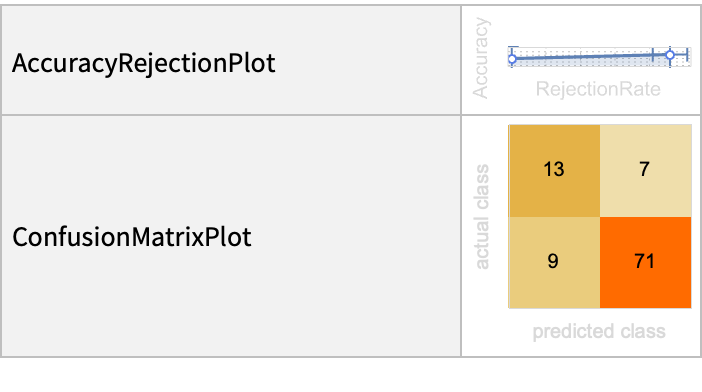### Applications (2)

Obtain a variety of statistics on a sample confusion matrix found in the Wikipedia entry for that topic: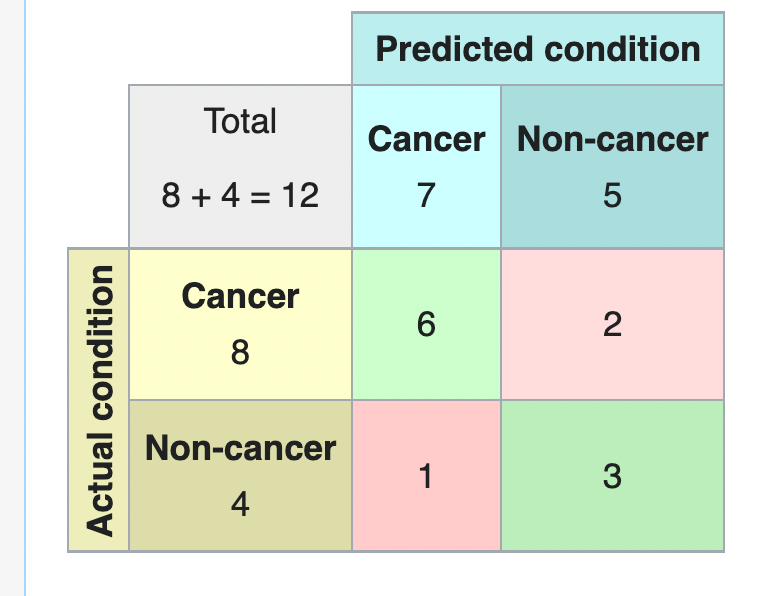In:=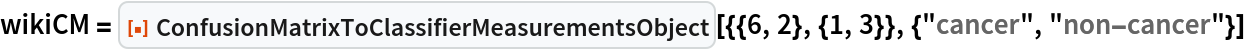Out=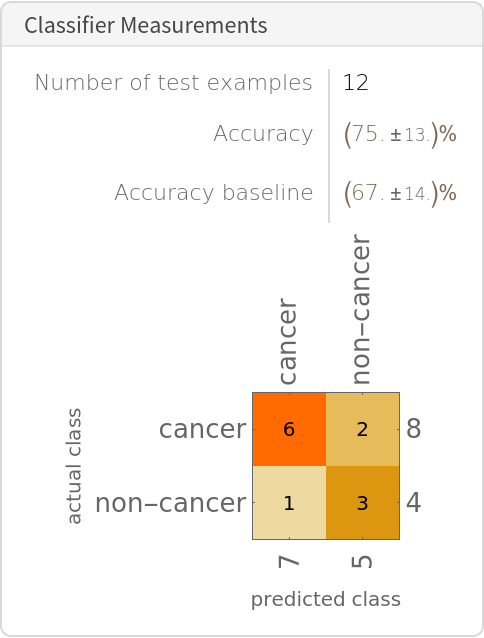In:=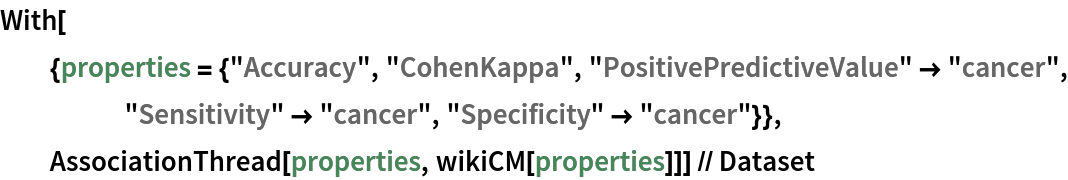Out=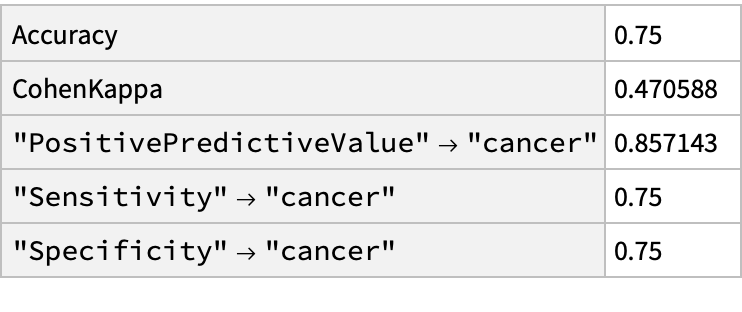Perform a Bayesian analysis with the data by computing the probability that a person who tests negative for cancer really does not have cancer:

 In:=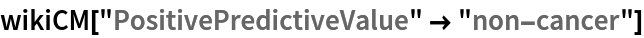Out=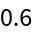Examine the accuracy and "ROCCurve" of a classifier in which one uses sex as a basis for determining if someone would survive the sinking of the Titanic:

 In:=Out=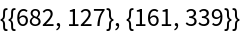In:=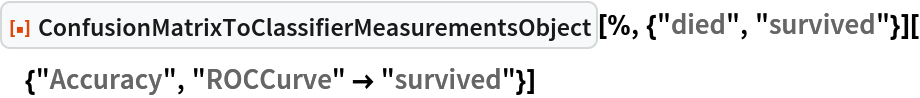Out=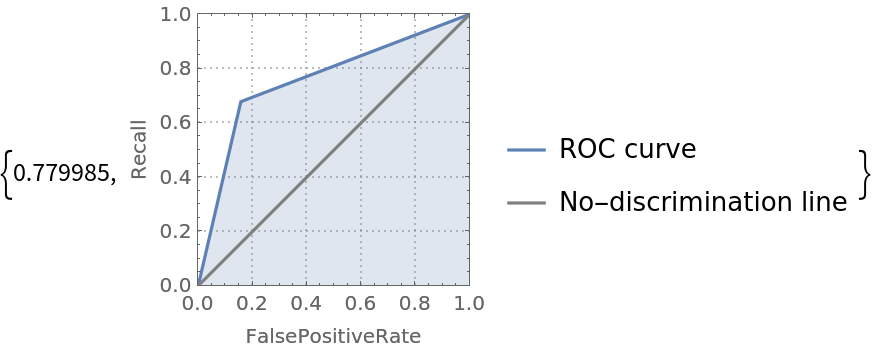### Possible Issues (5)

Some properties of a ClassifierMeasurementsObject will be Missing:

 In:=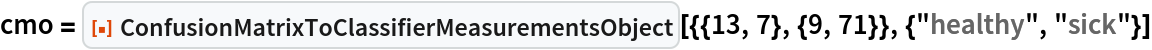Out=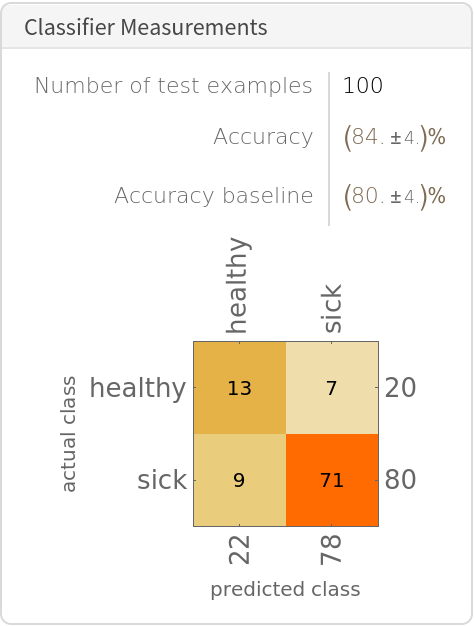In:=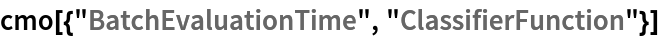Out=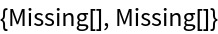The "ClassMeanCrossEntropy" property will take on infinite values:

 In:=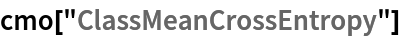Out=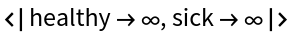Properties that yield show examples will not be well defined:

 In:=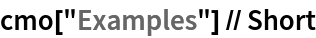Out=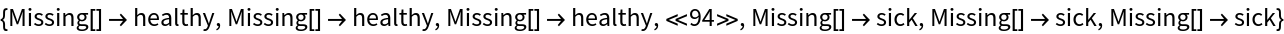If a row of the confusion matrix sums to zero, i. e. there are no examples of a certain class, an error message will be generated:

 In:=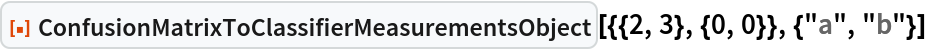Out=The function will work, however, if it has positive row sums but a column sum of the matrix is zero:

 In:=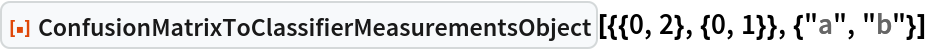Out=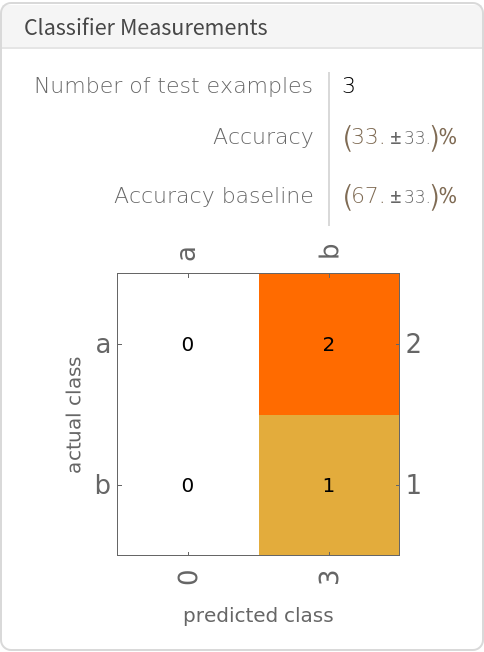### Neat Examples (4)

Create a composite confusion matrix from data that has been analyzed using cross-validation and determine the performance of the "NaiveBayes" classifier:

 In:=Out=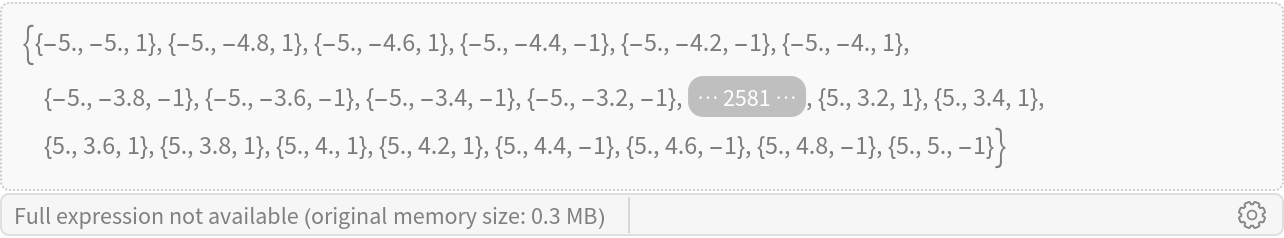Show a representative output from the CrossValidateModel resource function:

 In:=Out=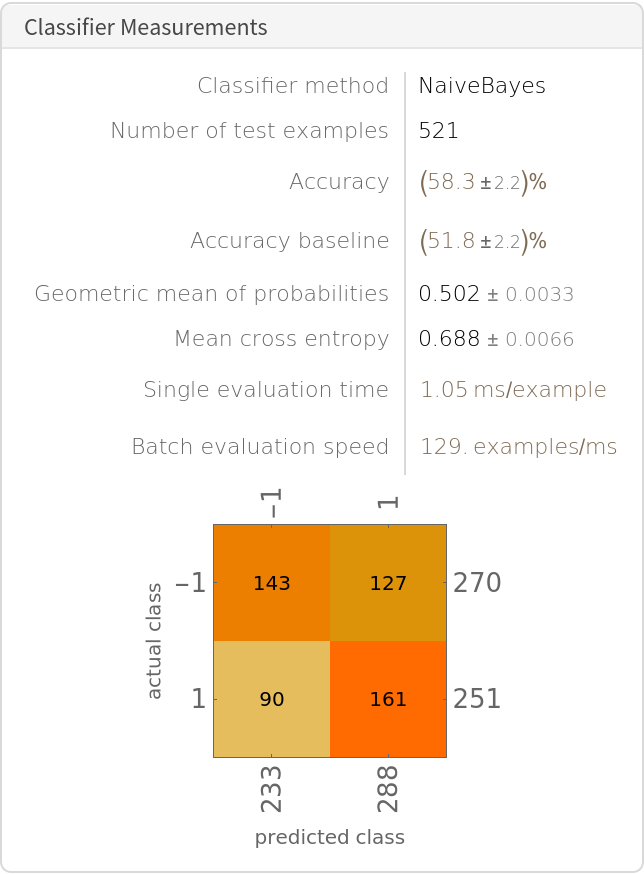Create a composite confusion matrix:

 In:=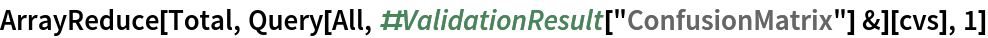Out=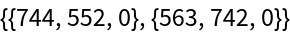Create the ClassifierMeasurementsObject:

 In:=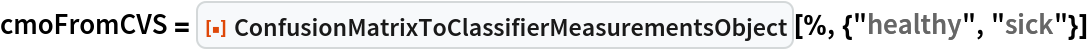In:=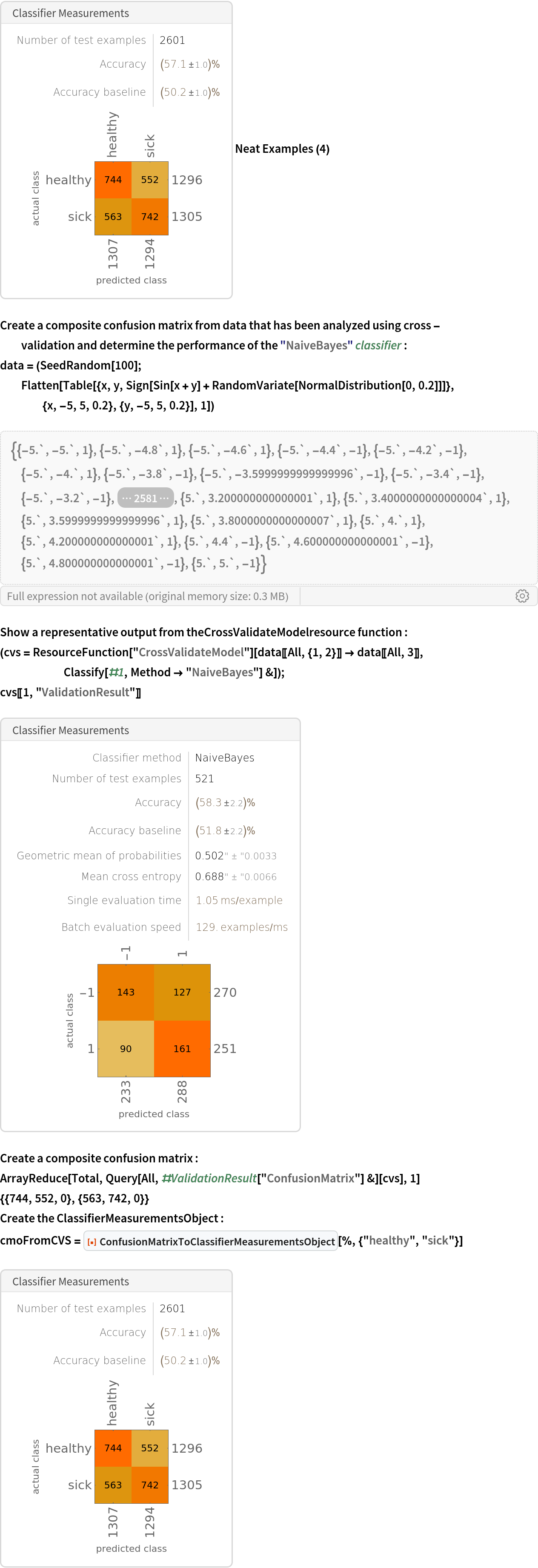Seth J. Chandler

## Version History

• 1.0.0 – 21 November 2022